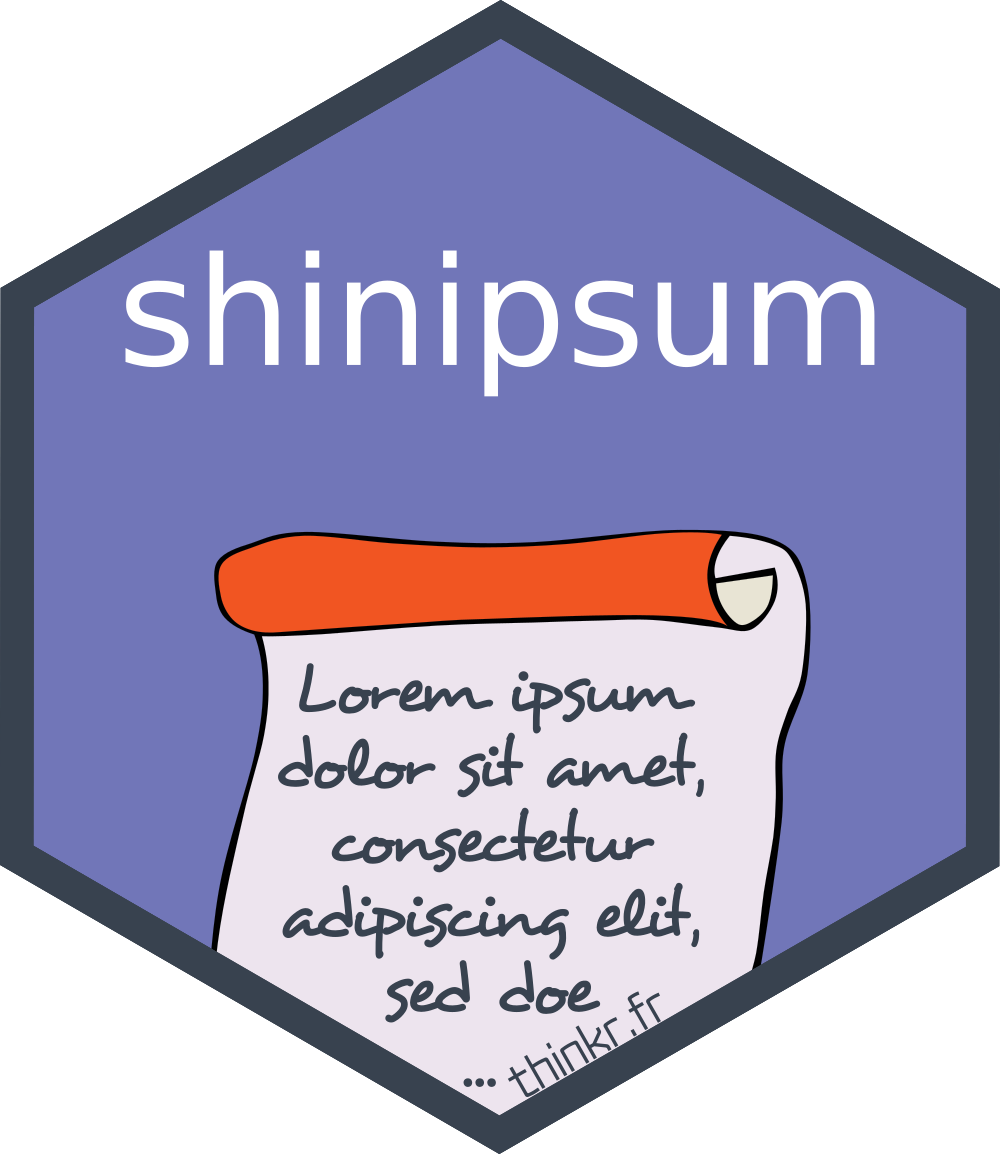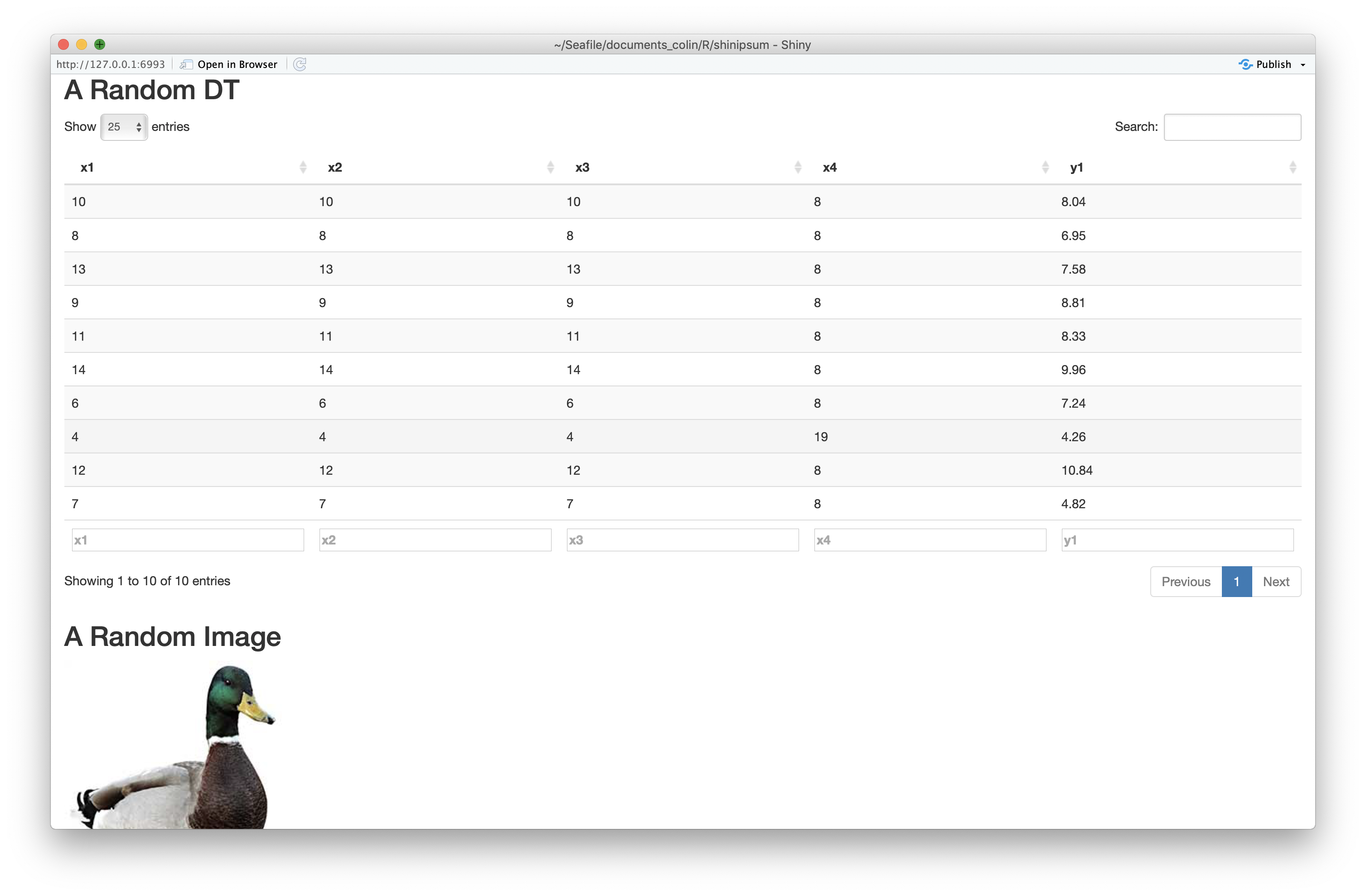# README.md In shinipsum: Lorem-Ipsum Helper Function for 'shiny' Prototyping# shinipsum

The goal of {shinipsum} is to provide random shiny elements for easiest shiny app prototyping, so that you can focus on building the frontend before building the backend.

The full documentation is in the {pkgdown} site: https://thinkr-open.github.io/shinipsum/

## Installation

You can install the dev version of shinipsum from GitHub with:

``````remotes::install_github("Thinkr-open/shinipsum")
``````

## Demo

Available examples:

``````library(shinipsum)
ipsum_examples()
#>  "01_navbar.R"
``````

You can run `{shinipsum}` demos with:

``````shiny::runApp(
ipsum_examples("01_navbar.R")
)
``````

## Available ipsums :

Note: {shinipsum} only load functions which are necessary to its internal job. If you want to customise an output or to use a renderXX / XXOutput, you’ll need to explicitely load the packages needed (for example, if you want to customise a dygraph, a ggplot, or use ggplotly).

### DataTable

`random_DT` takes 4 args :

• `nrow` & `ncol`: number of row and columns of the table
• `type` : random, numeric, character, numchar - the type of the columns
• `...` : args to be passed to `DT::datatable`

### Image

`random_image` returns a random image.

### Plot

`random_ggplot` takes one arg :

• `type` : Can be any of “random”, “point”, “bar”, “boxplot”,“col”, “tile”, “line”, “bin2d”, “contour”, “density”, “density_2d”, “dotplot”, “hex”, “freqpoly”, “histogram”, “ribbon”, “raster”, “tile”, “violin” and defines the geom of the ggplot. Default is “random”, and chooses a random geom for you.

Default theme is minimal.

As the return object is a `ggplot`, it can be enhanced like any other ggplot with `+`.

``````library(ggplot2)
random_ggplot(type = "col") +
labs(title = "Random plot") +
theme_bw()
``````

`random_ggplotly` calls the `ggplotly` function on a `random_ggplot`.

### Dygraph

`random_dygraph` returns a random dygprah. It takes one arg:

• `...`: arguments which are passed to the `dygraph()` function.

As the return object is a `dygraph`, it can be enhanced like any other dygraph.

``````library(dygraphs)
random_dygraph()  %>%
dyRangeSelector()
``````

### Print

`random_print` takes one arg:

• `type`: can be any of `"character", "numeric", "model", "table"`, and defines the type of print. Default is `"character"`.

### Table

`random_table` takes three args : `nrow`, `ncols` and `type`. See `random_DT`.

### Text

`random_text` takes one of these two args :

• `nchar` : lorem ipsum of `nchar` characters
• `nwords` : lorem ipsum of `nwords` characters

One of the two should be left `NULL`

## Example

Here is an example of using `{shinipsum}` to generate a random app:

``````library(shiny)
library(shinipsum)
library(DT)
ui <- fluidPage(
h2("A Random DT"),
DTOutput("data_table"),
h2("A Random Image"),
plotOutput("image", height = "300px"),
h2("A Random Plot"),
plotOutput("plot"),
h2("A Random Print"),
verbatimTextOutput("print"),
h2("A Random Table"),
tableOutput("table"),
h2("A Random Text"),
tableOutput("text")
)

server <- function(input, output, session) {
output\$data_table <- DT::renderDT({
random_DT(10, 5)
})
output\$image <- renderImage({
random_image()
})
output\$plot <- renderPlot({
random_ggplot()
})
output\$print <- renderPrint({
random_print("model")
})
output\$table <- renderTable({
random_table(10, 5)
})
output\$text <- renderText({
random_text(nwords = 50)
})
}
shinyApp(ui, server)
``````Please note that the ‘shinipsum’ project is released with a Contributor Code of Conduct. By contributing to this project, you agree to abide by its terms.

## Try the shinipsum package in your browser

Any scripts or data that you put into this service are public.

shinipsum documentation built on May 1, 2020, 1:07 a.m.